American Journal of Modern Energy
Volume 2, Issue 1, February 2016, Pages: 1-4

Radial Atomic Properties of Excited States for Beryllium Atom (1s2 2s ns) (1s)

Ruqaya Jabir Hadi1, Ali Abid Abojassim1, Laith Najam2

1Department of Physics, College of Science, Kufa Univ., Kufa, Iraq

2Department of Physics, College of Science, Mosul Univ., Mosul, Iraq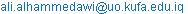(A. A. Abojassim)

Ruqaya Jabir Hadi, Ali Abid Abojassim, Laith Najam. Radial Atomic Properties of Excited States for Beryllium Atom (1s2 2s ns) (1s). American Journal of Modern Energy. Vol. 2, No. 1, 2016, pp. 1-4. doi: 10.11648/j.ajme.20160201.11

Received: April 9, 2016; Accepted: June 3, 2016; Published: June 17, 2016

Abstract: Some radial atomic properties of Be-atom in different excited states (1s2 2s 3s, 1s2 2s 4s, 1s2 2s 5s) (1s) have been obtained using two electron density function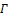(r1,r2) in order to solve Hartree-Fock equations using slater type orbitals using partitioning technique within the individual electronic shells of different configuration of Be-atom in position space. Radial expectations values for one electron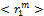and two electrons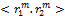, correlation coefficients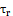, electron density at the nucleus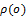, the nuclear magnetic shielding constant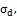and The diamagnetic susceptibility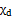have been calculated for these states of the same atom.

Keywords: Hartree-Fock-Roothaan Method, Slater Type Orbitals, Two Electron Density Function, Radial Expectation Values, The Nuclear Magnetic Shielding Constant

Contents

1. Introduction

The Hartree–Fock–Roothaan (HFR) is one of the approximated methods for solving multi electron systems problem and it is powerful method for studying atomic and molecular systems not only for ground state but also for excited states. In this literature the radial part of one electron basis is expanded in terms of slater type orbital (STOs) defined by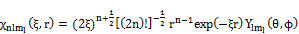(1)

Here,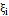> 0 is the orbital exponent. The quantity n occurring in eq. (1) is a positive principal quantum number of (STO). The determination of nonlinear parameters n andis very important for describing the atomic orbitals.

2. Theory

Most of the physically properties of a multi electron system can be calculated from the two-particle reduced density matrix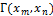, without reference to the wavefunction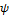. It is contains all the necessary information to compute the energy and many properties of the atoms. It can be gained by integrating the spin and spatial coordinates of all electrons except two electrons () from the N-particle density matrix, so the two-electron reduced density matrix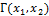given by [2, 3].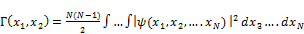(2)

Where=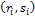combined space and spin variable with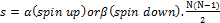represents the number of electron pairs which can be obtained by integrating the second-order reduced density matrix, so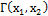is normalized to the number of independent electron pairs within the systemas [4,5].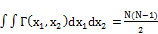(3)

The density for the individual electronic shells is obtained by partitioninginto its pair-wise components (i,j), where (i,j) labels the occupied normalized spin-orbitals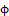in the HF description, the two electron density can be written as [6,7].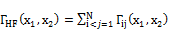(4)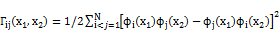(5)

For Be -atom the number of electron pairs is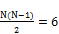(6)

3. Expectation Values

Expectation value for one-electronis determined by the following expression .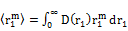(7)

Where m integer number (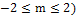. From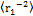leads to evaluate the correlation coefficient. The radial expectation value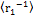leads to the electron-nuclear potential energy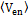and the nuclear magnetic shielding constant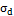, from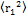, we determined the atomic diamagnetic susceptibility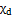,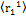represented the distance between nucleus and the electron.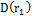is the one-electron radial density function which represents the probability density function to finding an electron at a distance between r1 and r1 + dr1 from the coordinate origin ( i.e nucleus). The single-electron radial density D(r1) is obtained from integration over two–electron radial density function D(r1, r2) and defined as [9,10],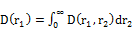(8)

Where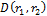is the two-electron radial density function and represents the probability density that one electron is located at a radius r1 and the other electron at a radius r2 simultaneously. The two-electron radial density function is given by .= ∫∫(r1,r2)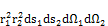(9)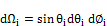Whereis the solid angle.

Two particle expectation value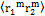is defined as .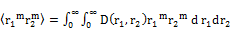(10)

3.1. Nuclear Magnetic Shielding Constant

The nuclear magnetic shielding constant is determined from the formula .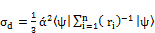(11)

Where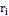is the distance from the nucleus to the electron in unit of atomic unit. and (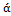) fine structure constant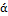=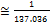in the atomic units the inner electronic shells shield the external ones thus giving a smaller effective nuclear charge for the outer electrons. the predominant contribution of the S atomic orbitals, and the decreasing relative contribution of the external orbitals as compared to the inner one[14,15].

3.2. The Diamagnetic Susceptibility

The magnetization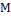of a substance is proportional to the magnetizing (external) magnetic field B, thus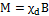. The proportionality factor is the magnetic susceptibility (). The vast majority of substances have negative magnetic susceptibility (are diamagnetic),or positive, which can be paramagnetic substances . The diamagnetic susceptibility is defined by relation:(12)

3.3. Electron Density at the Nucleus

The electron density at the nucleus can be evaluated using the following form .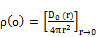(13)

3.4. Correlation Coefficients

The correlation coefficients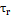are similar to those used in classical statistics.for two electron shell they are defined as .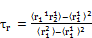(14)

This quantity is restricted by the value -1≤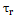≤ +1,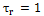(,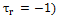means prefect positive (negative) correlation and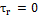means either non-correlated variables or for independent variables. For the atomic systems non-correlated variables means that the position vectors of any pairs of particles are orthogonal while independent variables means that the diagonal term of the two particle density matrix is the product of the one particle distribution functions. In these systemsfor inner shell (KαKβ )-shell for different excited states.

4. Results and Discussion

Different excited states (1s2 2s ns) (1s) of Be-atom where n=3,4,5 have been analyzed and some atomic properties have been calculated, the one electron expectation value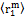, two electron expectation valuesthe nuclear magnetic shielding constant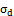, the diamagnetic susceptibility,the electron density at the nucleus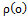and radial correlation coefficient,it should be mentioned that all numerical calculations have been performed in the Hartree-Fock frame work where in these systems there are six shells, the shells of state 1s2 2s 3s are (KαKβ, LαMβ LαMβ  KαLα, KαMβ, KαMβ, KβMβ ),the shells of state           1s2 2s 4s are (KαKβ, LαNβ KαLα, KβLα, KαNβ,KβNβ)while the shells of state 1s2 2s 5s are (KαKβ, LαOβ  KαLα, KβLα, KαOβ, KβOβ). Table (1) shows the calculated one electron expectation values for studied systems. Table (2) represents expectation values for two electrons, table (3) represents the nuclear magnetic shielding constant, table (4)shows the diamagnetic susceptibility, table (5) represents the electron density at the nucleus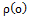and table (6) represents the radial correlation coefficient.

Table 1. One particle expectation value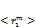for Be atom in different excited states for each individual electronic shell and for total system.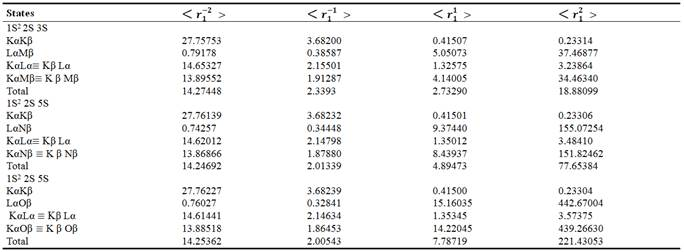Table 2. Two particles expectation values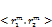for Be atom in different excited states for each individual electronic shell and for total system.Table 3. The Nuclear magnetic Shielding Constant for Be atom in different excited states for each individual electronic shell and for total system.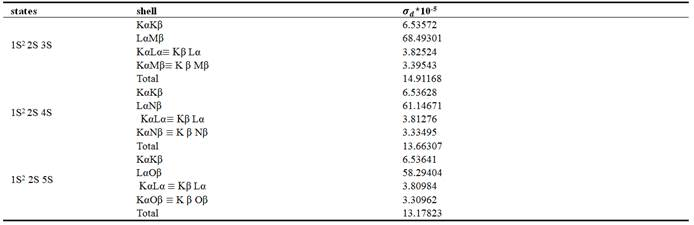Table 4. Diamagnetic Susceptibility for Be atom in different excited states for each individual electronic shell and for total system.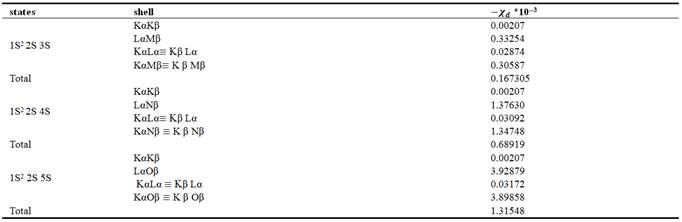Table 5. Electron density at the nucleus for Be atom in different excited states for each individual electronic shell and for total system.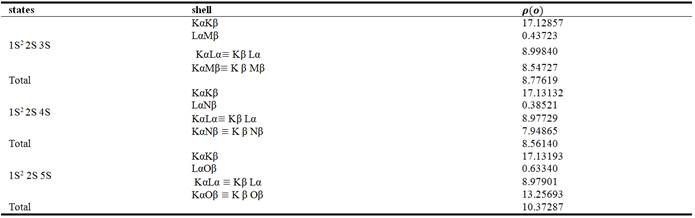Table 6. Correlation coefficients for Be atom in different excited states for each individual electronic shell and for total system.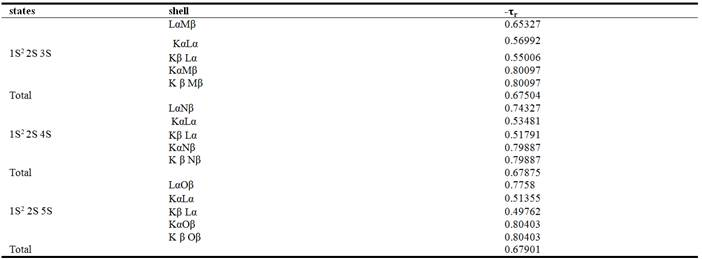5. Conclusion

This paper shows the effect of radial expectation valuesof Be-atom in its excited states (1s2 2s ns) where n=3,4,5 to calculate some atomic properties for these systems. When m= -1 this leads to calculate the nuclear magnetic shielding constant, when m = 1 this means the expectation value for the distance between an electron and the nucleus and also leads to determine the correlation coefficients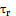and when m = 2 this leads to calculate The diamagnetic susceptibilityusing Hartree-Fock approximation.

References

1. T. Koga and Y. Kawata,J. Chemical Physics,Vol. 117, No. 20, 9133-9137 (2002).
2. T. Koga and H. Matsuyama, J. Chemical Physics, Vol. 120, No. 17, 7831- 7836 (2004).
3. S. Goedecker and C. J. Umrigar, J. Physical Review Letters, Vol. 81, No.4(1998) 866-868.
4. M. Fukuda and K. Fujisawa, arXiv:1010.4095,Vol.2 (2011).
5. K. E. Banyard and R. J. Mobbs, J. Chemical Physics, Vol. 75, No. 7 (1981) 3433-3442
6. T. Koga and Y. Kawata, J. Chemical Physics, Vol. 117, No. 20 (2002) 9133-9137.
7. C.Chen, J. The European Physical D, Vol.56, 303-309 (2010).
8. T. Koga and H. Matsuyama, J. Theor Chem Acc, Vol.115, 59–64 (2006).
9. H. Matsuyama and T. Koga, J. Computational And Applied Mathematics, Vol.233, 1584-1589 (2010).
10. T. Koga and H. Matsuyama, J. Theor Chem Acc,Vol.118,931–935(2007).
11. K. H. ALBayati, Ph.D, Thesis Leicester University, England (1986).
12. K.J.AL-Khafaji and A.F.Salman, J.Kufa Physics, Vol.4, No.1 (2012).
13. V. B. Mushkin and R.M. Aminova,J.Molecular Structure (Theochem),Vol.572 (2001) 185-191.
14. R. H. Romero, S. S. Gomez,J. Physics Letters A,Vol. 353 (2006) 190–193.
15. J. Penuelas, J. Liusia, B. Martınez, and J. Fontcuberta,J. Electromagnetic Biology And Medicine, Vol. 23, No. 2(2004) 97–112.
16. T. Koga and H. Matsuyama, J. Theor Chem Acc,Vol.115(2006) 59–64.
17. F.W.King and R. Dressel, J.Chem.Phys. Vol, 6449(1989).
18. K.E.Banyard and R.J. Mobb J. Chem.Phys. Vol. 75, No.7 (1981).
19. F.J.Galvez, E.Buendia and A.Sarsa J. Chem.Phys. Vol. 118, No.15 (2003).

 Contents 1. 2. 3. 3.1. 3.2. 3.3. 3.4. 4. 5.
Article ToolsAbstractPDF(217K)